## TONEMAP2

 Enhances the shadows and/or highlight regions in an image using a non-linear log or gamma function plus an extra processing function.

### EXAMPLES

(Processed With Q16 HDRI IM Compile)

 Note: Over time there have been changes to IM. Therefore, some examples now are better and some worse. So some arguments may need changing, but may not fix all the examples. Also -C sRGB or RGB will need swapping on current versions of IM.

 Example 1 -- variation in type Original Displayed As JPEG (Actual EXR Image Used) (source image web site)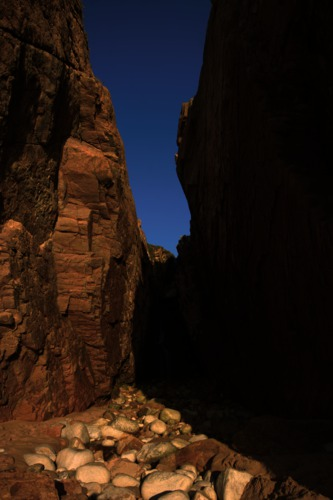Arguments: -t none -s -5 -h 10 -e -40 -S 0.5 Arguments: -t equalize -s 50 -h 20 -e -40 -S 0.5Arguments: -t space -s 0 -h 10 -e -40 -S 0.5 Arguments: -t retinex -s 20 -h 20 -e -40 -S 0.5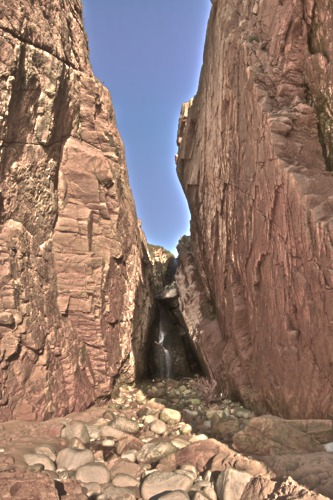Example 2 -- variation in type Original Displayed As JPEG (Actual EXR Image Used) (source image web site)Arguments: -t none -s 50 -h 0 -e -30 -S 0.5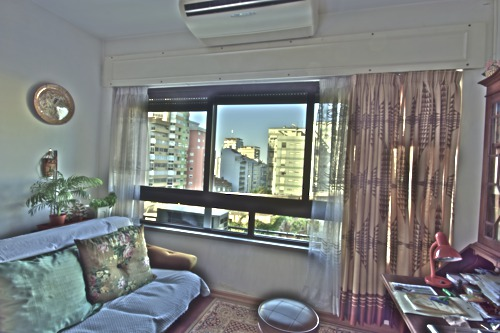Arguments: -t equalize -s 50 -h 0 -e -30 -S 0.5Arguments: -t space -s 50 -h 0 -e -30 -S 0.5Arguments: -t retinex -s 50 -h 0 -e -30 -S 0.5Example 3 -- variation in type Original Displayed As JPEG (Actual EXR Image Used) (source image web site)Arguments: -t none -s 50 -h 0 -e -30 -S 0.5Arguments: -t equalize -s 50 -h 0 -e -30 -S 0.5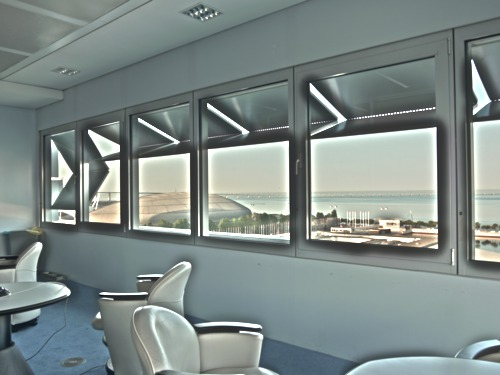Arguments: -t space -s 50 -h 0 -e -30 -S 0.5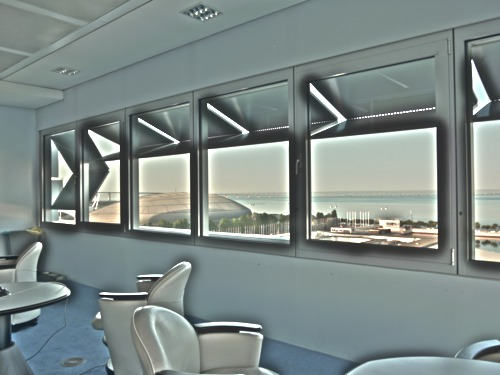Arguments: -t retinex -s 50 -h 0 -e -30 -S 0.5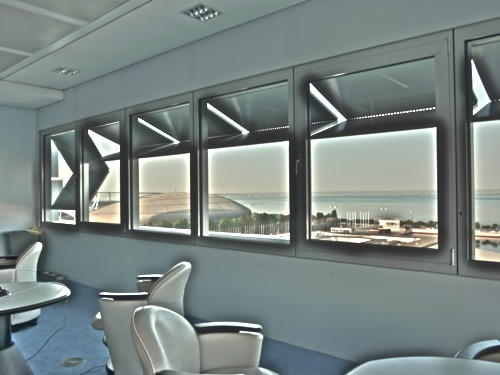Example 4 -- variation in type -- sRGB Original Displayed As JPEG (Actual HDR Image Used) (source image web site)Arguments: -t none -s -20 -h -10 -e -30 -S 0.5 -C sRGBArguments: -t equalize -s 30 -h 10 -e -30 -S 0.5 -C sRGB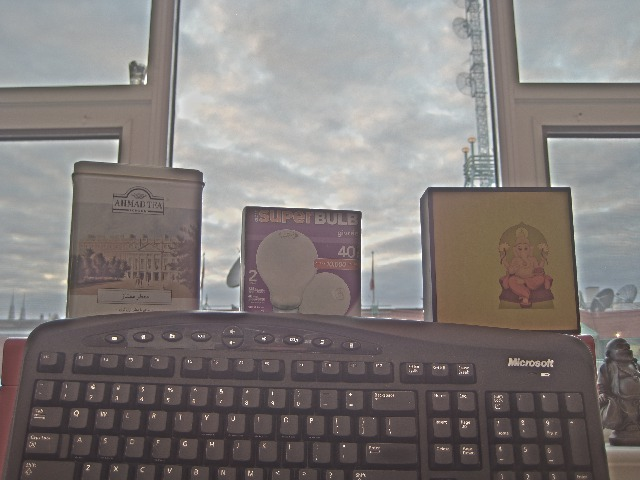Arguments: -t space -s -30 -h -10 -e -30 -S 0.5 -C sRGBArguments: -t retinex -s 30 -h 0 -e -30 -S 0.5 -C sRGB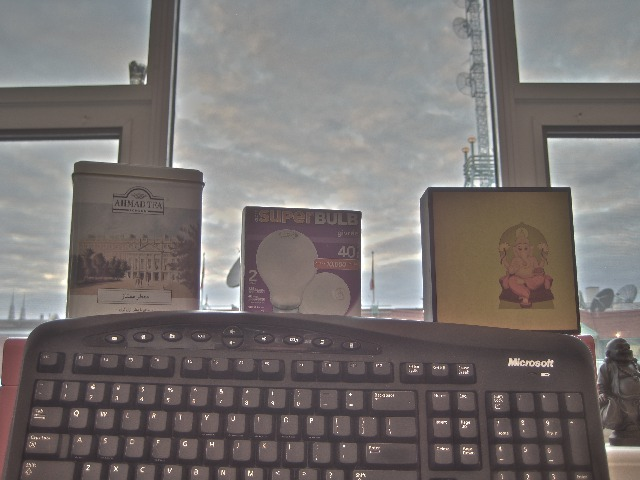Example 5 -- variation in type -- RGB Original Displayed As JPEG (Actual HDR Image Used) (source image web site)Arguments: -t none -s 10 -h 10 -e -30 -S 0.5 -C RGBArguments: -t equalize -s 30 -h 10 -e -30 -S 0.5 -C RGB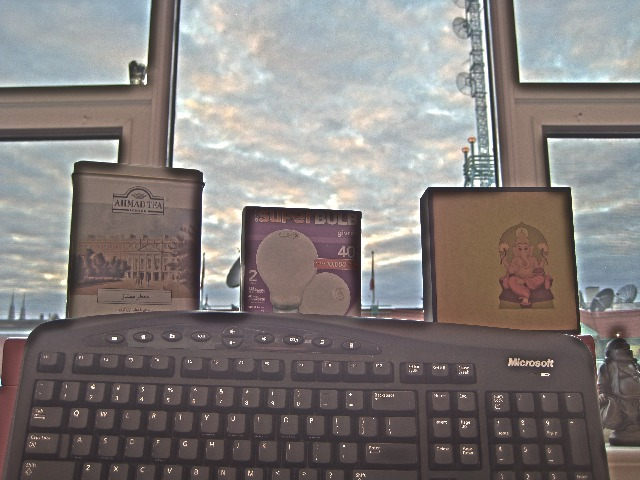Arguments: -t space -s 10 -h 10 -e -30 -S 0.5 -C RGBArguments: -t retinex -s 30 -h 30 -e -30 -S 0.5 -C RGB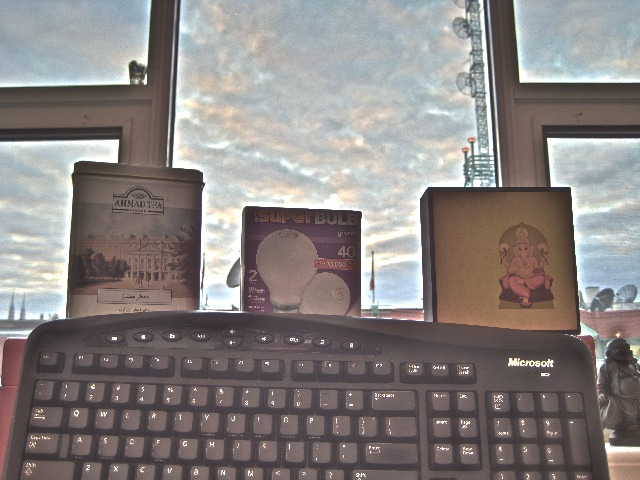Example 6 -- variation in type Original Displayed As JPEG (Actual HDR Image Used) (source image web site) (NOTE: Processed after .hdr bug fix at IM 6.7.2.0)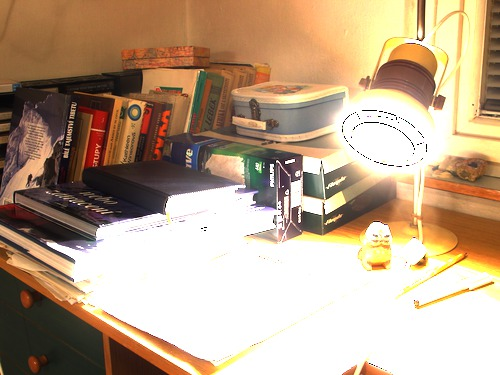Arguments: -t none -s 70 -h -15 -e -30 -S 0.6Arguments: -t retinex -s 70 -h 30 -e -30 -S 0.6Arguments: -t space -s 70 -h -15 -e -30 -S 0.6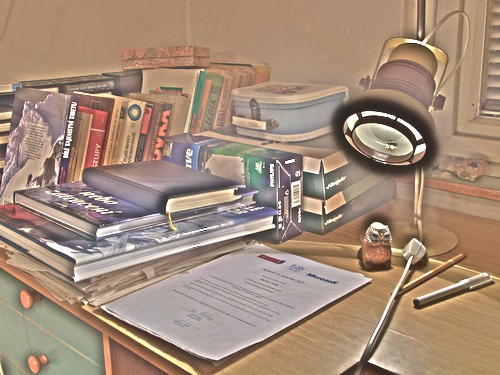What the script does is as follows for kind=equalize: Converts the image to HSB or HSL colorspace and separate channels Enhance the saturation channel Blurs intensity channel (B or L) Divides the intensity channel by the blurred intensity channel to form an edge image Enhance the edge image Applies the non-linear function followed by the -equalize to blurred intensity channel and then enhances it Multiplies the edge image with the processed blurred image as a modified intensity image Combine the original Hue channel with the modified Saturation channel and the modified intensity channel and converts back to RGB This is equivalent to the following IM commands for kind=equalize: if [ "\$cspace" = "none" ]; then cproc="" else cproc="-set colorspace \$cspace" fi if [ "\$alevel" = "yes" ]; then aproc="-auto-level" else aproc="" fi convert -quiet -regard-warnings "\$infile" -alpha off \$cproc \$aproc +repage "\$tmpA1" if [ "\$kind" = "log" ]; then amount=`convert xc: -precision 8 -format "%[fx:10^\$amount]" info:` proc0="-evaluate log \$amount" elif [ "\$kind" = "gamma" ]; then proc0="-gamma \$amount" fi if [ "\$sat" = "1" ]; then saturation="" else saturation="-evaluate multiply \$sat" fi ssign=`convert xc: -format "%[fx:sign(\$shadows)==-1?1:0]" info:` hsign=`convert xc: -format "%[fx:sign(\$highlights)==-1?1:0]" info:` mlo=0 mhi=100 plo=0 phi=100 if [ "\$shadows" != "0" -a \$ssign -eq 1 ]; then mlo=\$((-shadows)) elif [ "\$shadows" != "0" -a \$ssign -eq 0 ]; then plo=\$shadows fi if [ "\$highlights" != "0" -a \$hsign -eq 1 ]; then phi=\$((100+highlights)) elif [ "\$highlights" != "0" -a \$hsign -eq 0 ]; then mhi=\$((100-highlights)) fi if [ \$mlo -eq 0 -a \$mhi -eq 100 ]; then proc1="" else proc1="-level \$mlo,\$mhi%" fi if [ \$plo -eq 0 -a \$phi -eq 100 ]; then proc2="" else proc2="+level \$plo,\$phi%" fi convert \$tmpA1 -colorspace \$colormode -separate \ \( -clone 1 \$saturation \) \ \( -clone 2 -blur 0x\$blur \) \ \( -clone 2 -clone 4 +swap -compose divide -composite -brightness-contrast 0,\${edge} \) \ \( -clone 4 \$proc0 -equalize \$proc1 \$proc2 \) \ \( -clone 5 -clone 6 -compose multiply -composite \) \ -delete 1,2,4,5,6 -set colorspace \$colormode -combine -colorspace RGB \ \$outfile